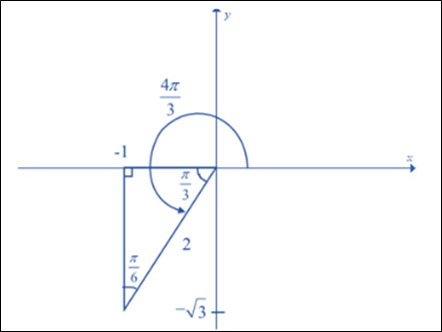# The value of the exact trigonometric ratio of the given radian angle: 4 π 3### Single Variable Calculus: Concepts...

4th Edition
James Stewart
Publisher: Cengage Learning
ISBN: 9781337687805### Single Variable Calculus: Concepts...

4th Edition
James Stewart
Publisher: Cengage Learning
ISBN: 9781337687805

#### Solutions

Chapter C, Problem 12E
To determine

## To calculate: The value of the exact trigonometric ratio of the given radian angle:   4π3

Expert Solution

The exact trigonometric ratio of the 4π3

sin4π3=32cosec4π3=23cos4π3=12sec4π3=2tan4π3=cot4π3=3

### Explanation of Solution

Given information:

4π3

Formula Used:

Trigonometric ratios are given by the following formula:

sinθ=yrcosecθ=rycosθ=xrsecθ=rxtanθ=yxcotθ=xy

Calculation:

The given value of radian is 4π3 . It is positive angle value that means the rotation is measured from the x axis as counter-clockwise direction.

Know that:

In one full rotation the angle is 2π . And the relation between the degree and radian is shown by below expression:

Now, rewrite the given angle in the standard position:

4π3=3π3+π34π3=π+π3

Thus, the given angle 4π3 measures angle half revolution plus π3 from the positive x axis.

This angle is drawn as below:Here, the triangle is fixed at terminal (x,y)=(1,3) and r=(1)2+(3)2=4=2

Therefore, trigonometric ratios are given as:

sin4π3=yr=32cosec4π3=ry=23cos4π3=xr=12sec4π3=rx=21=2tan4π3=yx=31=3cot4π3=xy=13=3

### Have a homework question?

Subscribe to bartleby learn! Ask subject matter experts 30 homework questions each month. Plus, you’ll have access to millions of step-by-step textbook answers!